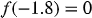1
37
views
18d
Problem

Problem 18d

Chapter 1: Functions and ModelsTextbook ExpertVerified Tutor
14 Nov 2021

Given information

Given that the graph of a function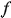is,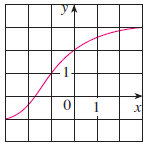Step-by-step explanation

Step 1.

Use the graph to estimate the value of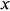at which function value is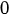.

From the graph we can see that the curve intersects-axis approximately at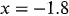.

Therefore,